A continuous linear operatorsuch that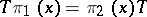, where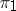andare mappings of a setinto two topological vector spaces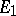andand. This concept is especially fruitful in the case whenis a group or an algebra andare representations of. The set of intertwining operators forms the space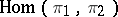, which is a subspace of the space of all continuous linear mappings fromto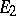. If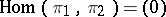and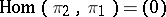, thenand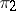are called disjoint representations. Ifcontains an operator that defines an isomorphism ofand, thenandare equivalent. If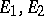are locally convex spaces, if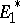and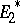are their adjoints, and ifand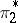are the representations contragredient toand, respectively (cf. Contragredient representation), then for any, the operator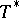is contained in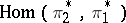. Ifandare finite-dimensional or unitary representations andis irreducible, thenadmits a subrepresentation equivalent toif and only if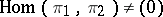. See also Intertwining number.